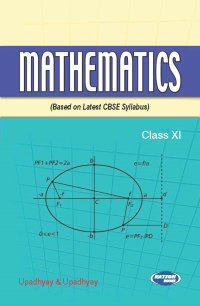•## Mathematics (CBSE) (Class - XI)

In Stock
Rs 525.00 Rs 473.00

#### CONTENTS

• Sets
• Relations and Functions

• Trigonometric Functions
• Principle of Mathematical Induction
• Complex Numbers
• Linear Inequalities
• Permutations and Combinations
• Binomial Theorem
• Sequence and Series
• Straight Lines
• Conic Sections
• Introduction to Three Dimensional Geometry
• Limits and Derivative
• Mathematical Reasoning
• Statistics
• Probability.

• Publisher : S.K. Kataria & Sons